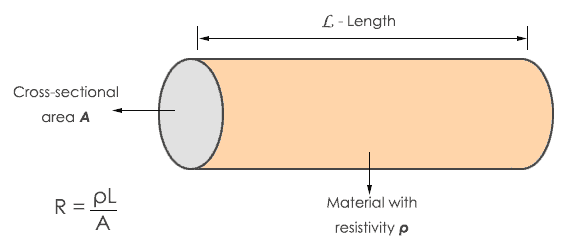# What is Electrical Resistance?

The electrical resistance of an electrical conductor is a measure of the difficulty to pass an electric current through that conductor. A conductor has free electrons randomly moving inside. When an electric potential is applied to it, the electrons collide with other atoms and molecules of the conductor. The atoms and molecules create the obstruction in the flow of electrons. This electrical opposition is called resistance.

Each and every material possesses the property of resistance because every conductor has atoms and the composition of materials is different. If the material has less electrical conductivity, it means the resistance of that material is high.

If we apply a pushing force or Electromotive force (E.M.F) across a conductor, electrons will move easily. This indicates that resistance is low. On the other hand, if we apply the same E.M.F. to an insulator it will produce little flow of electrons. Hence, the resistance of an insulator is high. So conductor like copper, gold, etc. has less resistance while insulators like wood, glass, etc. have higher resistance.

## Units of Resistance

Resistance is expressed in Ohms and is denoted by the Greek capital letter Ω (Omega). In many scenarios, we have to deal with materials having higher resistances and thus find the ohm for practical observations. For this reason, two larger quantities or multiples of ohm, that is to say, Kilo-Ohm (KΩ) and the Mega-Ohm (MΩ) are in general use.

1 KiloOhm = 1KΩ = 1000 Ω

1 Mega Ohm = 1MΩ = 1000 KΩ = 1,000,000 Ω

Here ‘K’ (Kilo) represent 1000 times which is equal to 10 3 and ‘M’ (Mega) represents Million times which is equal to 10 6.

## Laws of Resistance

The resistance of any material is affected by four physical quantities of the conductor. They are length, cross-sectional area, and resistivity and temperature. This resistance can be evaluated using the following four laws.### First Law

The value of resistance (R) is directly proportional to the length of the conductor. The law can be expressed by the equation R α L/A. It can be written as R= ρL/A. whereas ‘ρ’ (rho) is a constant of proportionality (also known as resistivity or specific resistance) and ‘L’ represents length (L) of the conductor.

The first law is represented by the statement R α L. This means, if the length of the conductor increases, the resistance also increases. In the same way, if the length of the conductor decreases, the resistance decreases.

### Second Law

From the formula R α L/A, the resistance is inversely proportional to the area of the conductor. The second law of resistance is given by the statement R α 1/A. This means if the length or area of the conductor increases the resistance decreases and vice versa.

### Third Law

Third law of resistance states that the conductor resistance depends upon the type or nature. This is specified as the resistivity of the material.

#### Resistivity (Specific Resistance)

It is defined as a measure of how strongly a material opposes the flow of electric current. The resistance of the conducting wire depends on the material of which wire is made. Every conductor has its own resistivity. Resistivity is denoted by the letter ρ.

According to our formula of resistance,

R = ρL/A

ρ = RA/L

Therefore, resistivity is directly proportional to the area of the conductor and inversely proportional to the length of the conductor. This means, increase in length decreases the resistivity and vice versa. And an increase in the area increases the resistivity of the material.

Unit of resistivity = ohms×m×m/m = ohms×m

### Fourth Law

The conductivity of the material will be more if the resistivity of that material is less and if resistivity is higher, the conductivity of the material will be less. So conductivity of the material is the inverse of resistivity. Conductivity is represented by σ.

This conductivity depends upon the temperature. Fourth law of resistance states that, if there is a rise in temperature it will increase the resistance of the conductor.

σ = 1/ρ

Unit of conductivity is 1/ohms×m or Siemens.

## Effects of temperature on Resistor

The resistance of the resistor changes with the change in temperature in surroundings. The temperature coefficient is generally taken to show the change. The temperature coefficient of the resistor is the amount of change in the resistor per degree Celsius change in temperature. It is denoted as α. If the resistor has a +ve temperature coefficient, then as the temperature increases the resistance in resistor also increases. If the resistor has –ve temperature coefficient, then as the temperature increases the resistance in the resistor decreases.

## Effects of pressure on Resistor

There is not much change in the resistivity of the conductor in small changes of pressure but only if the change in pressure is as high as 1 megabar i.e. 1Mbar. Thus, there is no notable change in resistor until this much pressure is applied. However, If the pressure is increased the resistivity of the resistor decreases.

Almost every electronic circuit consists of a resistor. No electric circuit can work without resistors because controlling of voltage is a most important thing of a circuit. Resistors are unsung heroes of electronics without which we cannot make some complex electronic systems.